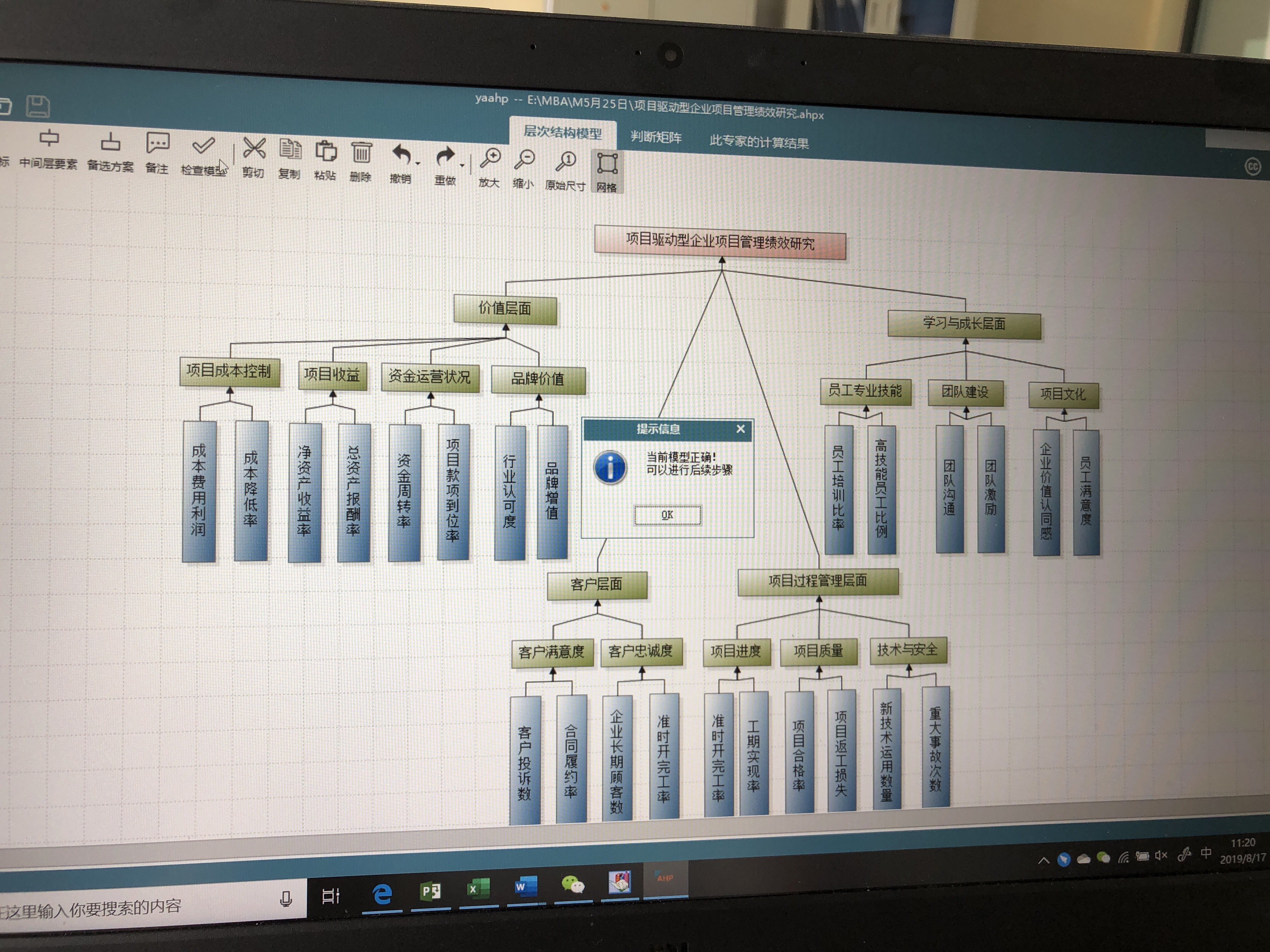• 构造判断矩阵的讲解(层次分析法)讲解判断矩阵和层次分析的关系。以及MATLAB的一些应用。
• 在AHP模型中，请一组专家对某一属性进行评价，根据专家的判断能力，给出判断力权值，再通过适当的数学处理方法，可得最优判断矩阵，并根据残差来确定所求判断矩阵是否达到精度...这是AHP中构造判断矩阵的一种新方法。
• 论文研究- 如何增加层次分析法判断矩阵的一致性.pdf, 一、前言 美国运筹学家T.L.Saaty教授提出的层次分析法(AHP)已在我国用于许多决策研究中,但在实际应用时,参加评判的专家或决策者对判断矩阵构造常感困难。...
• 层次分析法判断矩阵的填写方法、一致性检验的步骤、以及根据判断矩阵计算权重的方法

目录：
准则层判断矩阵怎么填写方案层判断矩阵怎么填写关于判断矩阵和一致矩阵的知识点补充一致性检验的步骤怎样通过判断矩阵去计算权重（三种方法），及相应的代码示例

准则层判断矩阵的填写：
填写准则层判断矩阵的目的是确定各准则（指标）所占的比重，填写好层次分析表的指标权重列，例如在选择最佳旅游地问题的指标景色、花费、居住、饮食、交通各自占比是多少，后续可以通过这些指标占比计算出每一个可选方案的总分。填表的方法是依据标度表，两两比较指标的重要程度，只需要比较10次就可以完成准则层判断矩阵的填写

方案层判断矩阵的填写
填写方案层判断矩阵的目的是给出，对于某一特定指标，它在各个可选方案的具体得分是多少，也就是给出层次分析表的每一横行的数据。方法是依据标度表，填写好判断矩阵。有几个评价指标，就需要填多少此方案层判断矩阵。知识点补充：
判断矩阵（正互反矩阵）
首先判断矩阵一定是一个方阵判断矩阵每一个数据 Aij表示与指标 j相比 i的重要程度当 i=j 时，两个指标相同，因此同等重要，记为1，因此判断矩阵的对角线元素为1每一个元素均大于零，且 Aij * Aji=1
在层次分析法中，我们构造的矩阵的均为判断矩阵
一致矩阵
矩阵首先满足判断矩阵的所有特点若判断矩阵满足 Aij * Ajk = Aik,直观的看就是矩阵的各行（各列）成倍数关系
注意点：在使用判断矩阵求权重之前，必须对其进行一致性检验

一致性检验的步骤：
第一步：计算一致性指标CI

C

I

=

λ

max

⁡

−

n

n

−

1

CI\,\,=\,\,\frac{\lambda _{\max}-n}{n-1}

第二步：查找对应的平均随机一致性指标RI第三步：计算一致性比例CR

C

R

=

C

I

R

I

CR\,\,=\,\,\frac{CI}{RI}

判断：如果CR<0.1,则可认为判断举证的一致性可以接受；否则需要对判断矩阵进行修改
一致性检验的MATLAB代码如下：
disp('请输入判断矩阵A')
A=input('A=');
[n,n] = size(A);
[V,D] = eig(A);%求出矩阵A的特征值和特征向量
Max_eig = max(max(D));%找到矩阵A的最大特征值
% 下面是计算一致性比例CR的环节 %
CI = (Max_eig - n) / (n-1);
RI=[0 0.0001 0.52 0.89 1.12 1.26 1.36 1.41 1.46 1.49 1.52 1.54 1.56 1.58 1.59];
%注意哦，这里的RI最多支持 n = 15
% 这里n=2时，一定是一致矩阵，所以CI = 0，我们为了避免分母为0，将这里的第二个元素改为了很接近0的正数
CR=CI/RI(n);
disp('一致性指标CI=');disp(CI);
disp('一致性比例CR=');disp(CR);
if CR<0.10
disp('因为CR<0.10，所以该判断矩阵A的一致性可以接受!');
else
disp('注意：CR >= 0.10，因此该判断矩阵A需要进行修改!');
end



通过判断矩阵求权重
方法一、算数平均法求权重
第一步：将判断矩阵按照列归一化（每一个元素除以器所在列的和）
第二步：将归一化的各列相加（按行求和）
第三步：将相加后得到的向量中的每个元素除以n即可得到权重向量
具体数学表达：
假设判断矩阵为下面这个矩阵A：

A

=

[

a

11

a

12

⋯

a

1

n

a

21

a

22

⋯

a

2

n

⋮

⋮

⋱

⋮

a

n

1

a

n

2

⋯

a

n

n

]

A=\left[ \begin{matrix} a_{11}& a_{12}& \cdots& a_{1n}\\ a_{21}& a_{22}& \cdots& a_{2n}\\ \vdots& \vdots& \ddots& \vdots\\ a_{n1}& a_{n2}& \cdots& a_{nn}\\ \end{matrix} \right]

那么算数平均法求得的权重向量为：

w

i

=

1

n

∑

j

=

1

n

a

i

j

∑

k

=

1

n

a

k

j

w_i=\frac{1}{n}\sum_{j=1}^n{\frac{a_{ij}}{\sum_{k=1}^n{a_{kj}}}}\,\,

MATLAB代码如下：
disp('请输入判断矩阵A')
A=input('A=');
[n,n] = size(A);

Sum_A = sum(A);   %sum函数默认是对矩阵的每一列进行累加，即按行求和
SUM_A = repmat(Sum_A,n,1);    %将Sum_A这个行向量，重复n行，重复一列
Stand_A = A ./ SUM_A;         %将矩阵A归一化，即每一个元素除以其所在列的和

disp('算术平均法求权重的结果为：');
disp(sum(Stand_A,2)./n)      %把归一化的矩阵的每一行累加，然后除以n,得到权重

方法二、几何平均法求权重
第一步：将A元素按照行相乘得到一个新的列向量
第二步：将新的列向量的每个分量开n次方
第三步：对该列向量进行归一化即可得到权重向量
假设判断矩阵为下面这个矩阵A：

A

=

[

a

11

a

12

⋯

a

1

n

a

21

a

22

⋯

a

2

n

⋮

⋮

⋱

⋮

a

n

1

a

n

2

⋯

a

n

n

]

A=\left[ \begin{matrix} a_{11}& a_{12}& \cdots& a_{1n}\\ a_{21}& a_{22}& \cdots& a_{2n}\\ \vdots& \vdots& \ddots& \vdots\\ a_{n1}& a_{n2}& \cdots& a_{nn}\\ \end{matrix} \right]

那么几何平均法求得的权重向量为：

w

i

=

(

∏

j

=

1

n

a

i

j

)

1

n

∑

k

=

1

n

(

∏

j

=

1

n

a

k

j

)

1

n

,

(

i

=

1

,

2

,

…

，

n

)

w_i=\frac{\left( \prod_{j=1}^n{a_{ij}} \right) ^{\frac{1}{n}}}{\sum_{k=1}^n{\left( \prod_{j=1}^n{a_{kj}} \right) ^{\frac{1}{n}}}},\left( i=1,2,…\text{，}n \right)

注意：每一种方法求得的权重和应该为1，由于四舍五入导致的误差可以忽略，一般结果保留四位小数
MATLAB代码如下：
disp('请输入判断矩阵A')
A=input('A=');
[n,n] = size(A);  %获得矩阵A的行和列的大小

Prduct_A = prod(A,2);       %把矩阵A的每一行累乘，即按照列累乘
Prduct_n_A = Prduct_A .^ (1/n);   %将新的列向量的每个分量开n次方
disp('几何平均法求权重的结果为：');
disp(Prduct_n_A ./ sum(Prduct_n_A))  %对该列向量进行归一化即可得到权重向量

方法三、特征值法求权重（常用）
知识点提醒：一致矩阵有一个特征值为n,其余特征值均为0，并且当矩阵的特征值为n时，其对应的特征向量为

k

[

1

a

11

,

1

a

12

,

…

,

1

a

1

n

]

T

,

(

k

≠

0

)

k\left[ \frac{1}{a_{11}},\frac{1}{a_{12}},…,\frac{1}{a_{1n}} \right] ^T,\left( k\ne 0 \right)

第一步：求出矩阵A的最大特征值和以及其对应的特征向量
第二步：对求出的特征向量进行归一化即可得到所求的权重
MATLAB代码如下：
disp('请输入判断矩阵A')
A=input('A=');

%求矩阵A的全部特征值，构成对角阵D，求A的特征向量构成V的列向量（V的每一列都是D中与之相同列的特征值的特征向量）
[V,D] = eig(A);
Max_eig = max(max(D));   %求出矩阵A的最大的特征值
[r,c]=find(D == Max_eig , 1);  %返回最大特征值所在的行和列，其中C记录所在列
disp('特征值法求权重的结果为：');
disp( V(:,c) ./ sum(V(:,c)) )  %对最大特征值对应的特征向量进行归一化处理

友情提示：在比赛当中，建议三种方法全部列出来，但仅适用特征值法求得的权重结果进行计算

更多有关与层次分析法的经典获奖论文，以及建模论文模板等资料关注公众号，回复，“层次分析法”，即可免费领取！！！展开全文• 然后用以下程序就好了：%层次分析法的matlab程序 %%%%diertimoxingyiclc,cleardisp('输入判断矩阵');% 在屏幕显示这句话A=input('A=');% 从屏幕接收判断矩阵[n,n]=size(A);% 计算A的维度，这里是方阵，这么写不太好x...

该楼层疑似违规已被系统折叠 隐藏此楼查看此楼
先确定判断矩阵；
然后用以下程序就好了：
%层次分析法的matlab程序 %%%%diertimoxingyi
clc,clear
disp('输入判断矩阵');% 在屏幕显示这句话
A=input('A=');% 从屏幕接收判断矩阵
[n,n]=size(A);% 计算A的维度，这里是方阵，这么写不太好
x=ones(n,100);% x为n行100列全1的矩阵
y=ones(n,100);% y同x
m=zeros(1,100);% m为1行100列全0的向量
m(1)=max(x(:,1));% x第一列中最大的值赋给m的第一个分量
y(:,1)=x(:,1);% x的第一列赋予y的第一列
x(:,2)=A*y(:,1);% x的第二列为矩阵A*y(:,1)
m(2)=max(x(:,2));% x第二列中最大的值赋给m的第二个分量
y(:,2)=x(:,2)/m(2);% x的第二列除以m(2)后赋给y的第二列
p=0.0001;i=2;k=abs(m(2)-m(1));% 初始化p，i，k为m(2)-m(1)的绝对值
while k>p% 当k>p是执行循环体
i=i+1;% i自加1
x(:,i)=A*y(:,i-1);% x的第i列等于A*y的第i-1列
m(i)=max(x(:,i));% m的第i个分量等于x第i列中最大的值
y(:,i)=x(:,i)/m(i);% y的第i列等于x的第i列除以m的第i个分量
k=abs(m(i)-m(i-1));% k等于m(i)-m(i-1)的绝对值
end
a=sum(y(:,i));% y的第i列的和赋予a
w=y(:,i)/a;% y的第i列除以a
t=m(i);% m的第i个分量赋给t
disp('权向量:');disp(w);% 显示权向量w
disp('最大特征值:');disp(t);% 显示最大特征值t
%以下是一致性检验
CI=(t-n)/(n-1);% t-维度再除以维度-1的值赋给CI
RI=[0 0 0.52 0.89 1.12 1.26 1.36 1.41 1.46 1.49 1.52 1.54 1.56 1.58 1.59];% 计算的标准
CR=CI/RI(n);% 计算一致性
if CR<0.10
disp('此矩阵的一致性可以接受!');
disp('CI=');disp(CI);
disp('CR=');disp(CR);
else
disp('此矩阵的一致性不可以接受!');
end

展开全文• 建立判断矩阵 层次分析法的一个重要特点就是用两两重要性程度之比的形式表示出两个方案的相应重要性程度等级。 4.计算层次单排序的权向量和一致性检验 对于每一个成对比较阵计算最大特征根及对应特征向量，利用一致...
一、引言
供应商管理，是在新的物流与采购经济形势下，提出的管理机制。供应链管理环境下的客户关系是一种战略性合作关系，提倡一种双赢机制。从传统的非合作性竞争走向合作性竞争、合作与竞争并存是当今企业关系发展的一个趋势。因此供应商选择对企业采购与供应工作具有直接的关键的影响。一个好的供应商能够在物料或服务的质量、成本等各个方面满足企业的需求，一个不合格的供应商在这些方面会给企业带来非常严重的问题与后果。无论是在传统的采购与供应管理中，还是在现在的供应链管理中，供应商选择都是关键环节，选择一个卓越的供应商，是企业的一项关键职能。
供应商选择的一般原则是：资金力量较强，产品质量较好，价格合理，服务质量好。所以本文的评价指标主要考虑质量、价格、交货、服务和提前期这五个指标。对这五个评价指标的具体描述如下：
产品质量：指供应商的产品满足企业需求的程度。一般用合格产品占总产品的比重计算，该指标越大越好，是“效益”型指标。
产品价格：指企业采购的每一单位产品所付出的成本，该指标越小越好，是“成本”型指标。
交货：指供应商及时满足企业定单的程度。一般用及时交货的定单数占总定单数的比例来表示，该指标值越大越优，是“效益”型指标。
服务：指供应商在交货后在产品安装、维护等方面满足企业的程度，该指标值越大越好，为“效益”型指标。
交货提前期：指企业发出定单到收到定货之间的时间，对于需求方来说，交货提前期越短越好，是“成本”型指标。
二、方法介绍
层次分析法首先将整个系统划分为目标、准则和方案三个层次，然后对方案进行相互比较，运用矩阵形式判断作相对评价，最后进行综合评价，排出各方案的优劣次序。具体做法可分为五个步骤：
1.明确目标
2.建立层次结构
在深入分析实际问题的基础上，将有关的各个因素按照不同属性自上而下地分解成若干层次，同一层的诸因素从属于上一层的因素或对上层因素有影响，同时又支配下一层的因素或受到下层因素的作用。最上层为目标层，通常只有1个因素，最下层通常为方案或对象层，中间可以有一个或几个层次，通常为准则或指标层。当准则过多时应进一步分解出子准则层。
3.建立判断矩阵
层次分析法的一个重要特点就是用两两重要性程度之比的形式表示出两个方案的相应重要性程度等级。
4.计算层次单排序的权向量和一致性检验
对于每一个成对比较阵计算最大特征根及对应特征向量，利用一致性指标、随机一致性指标和一致性比率做一致性检验。若检验通过，特征向量(归一化后)即为权向量：若不通过，需重新构追成对比较阵。
5.进行总层次排序
计算最下层对目标的组合权向量，并根据公式做组合一致性检验，若检验通过，则可按照组合权向量表示的结果进行决策，否则需要重新考虑模型或重新构造那些一致性比率较大的成对比较阵。
应用这种方法，决策者通过将复杂问题分解为若干层次和若干因素，在各因素之间进行简单的比较和计算，就可以得出不同方案的权重，为最佳方案的选择提供依据。
三、建立模型
1.明确目标：选择理想的供应商。
2.建立层次结构模型：该结构图包括目标层，准则层，方案层。
4.计算层次单排序的权向量：W=（0.263，0.475，0.005，0.090，0.110）。CR=0.016＜0.1通过一致性验证。
同样求第3层(方案)对第2层每一元素(准则)的权向量：
w1=(0.595，0.277，0.129)，w2=（0.082，0.236，0.682），w3=（0.429，0.429，0.142），w4=（0.633，0.193，0.175），w5=（0.166，0.166，0.668）。CR1=0.05，CR2=0.002,CR3=0，CR4=0.009，CR5=0，均＜0.1，通过一致性检验。
5.进行总层次排序
P1对总目标的权值为：0.595×0.263+0.082×0.475+0.429×0.055+0.663×0.099+0.166×0.110=0.3同理P2、P3 对总目标的权值分别为：0.246，0.456 决策层对总目标的权向量为：{0.3，0.246，0.456}，CR=0.015＜0.1层次总排序通过一致性检验。因此最佳供应商为P3。
四、小结
本文运用层次分析法对供应链管理中的供应商选择进行了评价，充分考虑了供应商的质量、成本、交货、服务和提前期，综合考虑各个供应商的优劣势，以及该层对上层的影响，选择了一个最优的供应商。随着供应链思想的普遍应用，供应商的选择显得越来越重要，因此本文具有一定的现实意义。
文末资源：层次分析法常用软件，破解版yaahp软件，中间层个数可达99个，方案层个数可达99个，免费版的中间层、方案层个数只有3个哦，内含教程，永久使用：（浏览器输入/点击网址获取）
层次分析法之yaahp软件，中间层、方案层99个，内含教程，提供技术支持！
https://k.ruyu.com/l8veTM3e
下图是我做的架构：展开全文• 利用粗糙集中属性约简的思想来确定各属性...在此基础上将客观矩阵和利用层次分析法确定的主观矩阵相结合，提出了一种组合矩阵，通过计算组合矩阵的权重来进行决策。实例表明该方法是切实可行、有效的，能提高决策精度。
• 层次分析法原理简单，matlab实现起来也较容易。 对于matlab新手而言，主要在判断矩阵未通过一致性检验的时候，需要重新输入，重新计算。 clc; clear;% 清除所有命令窗口，清除所有变量 while true %无条件进入循环 A...
层次分析法原理简单，matlab实现起来也较容易。 对于matlab新手而言，主要在判断矩阵未通过一致性检验的时候，需要重新输入，重新计算。
clc;
clear;% 清除所有命令窗口，清除所有变量
while true %无条件进入循环
A=input('请输入判断矩阵A=');
[m,n]=size(A);                     %获取指标个数
RI=[  0	 0	 0.58 	0.90	1.12	1.26	1.36	1.41	1.46];
[V,D]=eig(A);                      %求判断矩阵的特征值和特征向量，V特征值，D特征向量；
tz=max(D);
B=max(tz);                         %最大特征值
[row, col]=find(D==B);             %最大特征值所在位置
C=V(:,col);                        %对应特征向量
CI=(B-n)/(n-1);                    %计算一致性检验指标CI
CR=CI/RI(1,n);
if CR<0.10
disp('CI=');disp(CI);
disp('CR=');disp(CR);
disp('对比矩阵A通过一致性检验，各向量权重向量Q为：');
break;

else
disp('对比矩阵A未通过一致性检验，需对对比矩阵A重新构造');
continue;
end

end

Q=zeros(n,1);
for i=1:n
Q(i,1)=C(i,1)/sum(C(:,1)); %特征向量标准化
end
Q  %最后输出权重值

展开全文• ## 层次分析法（AHP）详细步骤

万次阅读 多人点赞 2019-01-07 13:01:10
构造判断矩阵 层次分析法构造判断矩阵的方法是一致矩阵法，即：不把所有因素放在一起比较，而是两两相互比较；对此时采用相对尺度，以尽可能减少性质不同因素相互比较的困难，以提高准确度。 判断矩阵 aija_{ij}...数学建模
• 第一讲：层次分析法 模型介绍 评价类问题可用打分解决 根据权重表格计算得分 一个小小的总结 一道引出层次分析法的例题 还记得这张权重表格吗？ 直接问权重的弊端 分而治之的思想 层次分析...数学建模
• 针对不具备专家群判实力的个人或团体在用层次分析法（AHP）进行决策时判断矩阵构造难的问题，提出“差标度法”合理确定标度，用这种方法构造判断矩阵在不违背人的主观意愿的前提下一次满足一致性，极大地简化了...
• AHP层次分析法代码+文档，文档非常详细，适合初学者使用，构造判断矩阵的讲解(层次分析法)，代码可正常运行
• 在一致性修正过程中,为了避免模糊层次分析法 (AHP)可能引发的歧义,提出了一种同步一致性算法和基于矩阵一致性指标的多判断矩阵合成方法。结合模糊判断矩阵构造和一致性检验2个步骤,利用人机交互的特点,在构造模糊...
• 构造判断矩阵（成对比较阵）并赋值4.层次单排序（计算权向量）与检验（一致性检验）计算权向量一致性检验5.层次总排序（组合权向量）与检验（一致性检验）6.结果分析7.层次分析法的优缺点层次分析法的代码实现...算法 matlab
• ## 层次分析法

万次阅读 多人点赞 2018-10-26 11:08:49
层次分析法（Analytic Hierarchy Process ，简称 AHP ）是对一些较为复杂、较为模糊的问题作出决策的简易方法，它特别适用于那些难于完全定量分析的问题。它是美国运筹学家T. L. Saaty 教授于上世纪 70 年代初期提出...
• ## AHP层次分析法

万次阅读 多人点赞 2014-02-21 01:07:12
在比赛中，我们运用了层次分析法（AHPAnalytic Hierarchy Process）进行建模，好不容易理解了这一方法的思想，在自己的博客里记录一下，希望可以帮助初次接触层次分析法的人，更快地理解这一的整体思想，也利于...数学建模
• 为了改进由层次分析法中的主观判断矩阵所引起的评价结果准确度的不足，借助于粗糙集理论中的属性依赖度定义，构造出属性间相对依赖的客观判断矩阵，在此基础上将该客观判断矩阵层次分析法中的主观判断矩阵相结合，...粗糙集
• 构造判断(成对比较)矩阵 第二层A的各个因素对目标层Z的成对比较矩阵（专家打分） 说明C2的重要性是C1的两倍 第三层B的各个因素A1，A2……A5的成对比较矩阵分别如下： 层次单排序及其一致性检验 ...python
• 为了更合理地确定影响煤与瓦斯突出因素的权重,在层次分析法判断矩阵构造上,论文主要用三标度法对传统的九标度法进行改进。利用改进的层次分析法确定影响煤与瓦斯突出各因素的权重,用隶属度构造了单因素模糊判别...
• 由于传统的煤炭资源评价方法很难对矿井资源储量这一定性问题进行定量评价,运用层次分析法,通过对影响资源储量的诸多因素建立层次结构模型,构造判断矩阵,对通过一致性检验的权值进行重要度因果分析,最终得出组合权值,...
• 针对传统攻击树模型在计算攻击事件发生概率时未考虑各安全属性权值的不足，设计了一种基于层次分析法的攻击树模型。在计算攻击事件发生概率时，首先给每个叶节点赋予不同安全属性；然后根据攻击者意图和系统特征比较...攻击树
• Python实现“层次分析法”及“自调节层次分析法” 假设我们遇到如下问题： ①对于M个方案，每个方案有N个属性...层次分析法的基本思想是将复杂问题分为若干层次和若干因素，在同一层次的各要素之间简单地进行比较判断算法 python
• 0. 层次分析法简介 层次分析法（Analytic Hierarchy Process，简称AHP）主要是对于定性的决策问题进行定量化分析的方法。举个例子，在日常生活中，我们经常需要进行感性的判断，比如报高考志愿，感觉清华北大都很好...一致性检验...